﻿ 动力系统中燃气轮机特性仿真与计算方法研究 Research on the Mode Calculation and Characteristic Modularization of the Gas Turbine in the Power System

Dynamical Systems and Control
Vol. 08  No. 01 (2019 ), Article ID: 28070 , 8 pages
10.12677/DSC.2019.81003

Research on the Mode Calculation and Characteristic Modularization of the Gas Turbine in the Power System

Guang Xu1, Baihui Xu1, Qianchao Liang2, Hen Qin2

1Naval Equipment Section of the East China Sea Fleet, Ningbo Zhejiang

2College of Naval Architecture and Power, Naval University of Engineering, Wuhan HubeiReceived: Nov. 26th, 2018; accepted: Dec. 11th, 2018; published: Dec. 18th, 2018ABSTRACT

In this paper, a joint power system control is taken as the research object, which solves the complicated logical relationship of the remote control system, the logical reasoning structure, and the control of the Ethernet system. The control system simulation is based on the system dynamics, nonlinear causes and calculation methods. It uses a quasi-steady state method, a volumetric method, and a wave action method to calculate a nonlinear system. It is pointed out that the dynamic characteristics of the power system will ultimately affect the design performance of the whole power plant.

Keywords:Power System Plant (CODOG), Modularization, Simulation

1海军92910部队，浙江 宁波

2海军工程大学，湖北 武汉1. 前言

2. 系统建模方法介绍

3. 系统数学模型仿真与推进系统的性能分析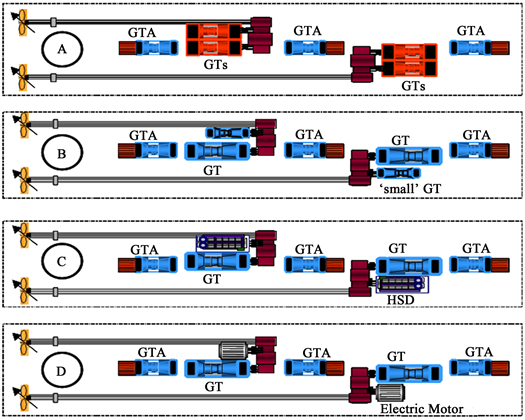Figure 1. Power system model

1) 惯性环节的微分方程式

${I}_{1}{\omega }_{1}\frac{\text{d}{\omega }_{1}}{\text{d}t}={N}_{eLT}-{N}_{eLC}$ (3.1.1)

${I}_{2}{\omega }_{2}\frac{\text{d}{\omega }_{2}}{\text{d}t}={N}_{eHT}-{N}_{eHC}$ (3.1.2)

${I}_{3}{\omega }_{3}\frac{\text{d}{\omega }_{3}}{\text{d}t}={N}_{ePT}-{N}_{eN}$ (3.1.3)

${V}_{1}\frac{\text{d}{\rho }_{2}}{\text{d}t}={G}_{LC}{g}_{LC}-{G}_{HC}$ (3.1.4)

${V}_{2}\frac{\text{d}{\rho }_{3}}{\text{d}t}={G}_{HC}{g}_{HC}\left(1+f\right)-{G}_{HT}$ (3.1.5)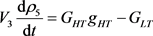( 3.1.6 )

${V}_{4}\frac{\text{d}{\rho }_{6}}{\text{d}t}={G}_{LT}{g}_{LT}-{G}_{PT}$ (3.1.7)

${M}_{s}\frac{{d}_{{\nu }_{s}}}{{d}_{\tau }}=±{T}_{P}-R$ (3.1.8)

2) 部件的特性方程组

${\tau }_{LC}=\frac{{T}_{2}^{\ast }}{{T}_{1}^{\ast }}={f}_{1}\left(\frac{{G}_{LC}\sqrt{{T}_{1}^{\ast }}}{{P}_{1}^{\ast }},\frac{{n}_{1}}{\sqrt{{T}_{1}^{\ast }}}\right)$ (3.1.9)

${\pi }_{LC}^{\ast }=\frac{{P}_{2}^{\ast }}{{P}_{1}^{\ast }}={f}_{2}\left(\frac{{G}_{LC}\sqrt{{T}_{1}^{\ast }}}{{P}_{1}^{\ast }},\frac{{n}_{1}}{\sqrt{{T}_{1}^{\ast }}}\right)$ (3.1.10)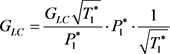( 3.1.11 )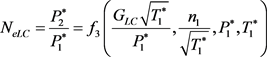( 3.1.12 )(3.1.13)

${\epsilon }_{HC}^{\ast }=\frac{{P}_{3}^{\ast }}{{P}_{2}^{\ast }}={f}_{5}\left(\frac{{G}_{HC}\sqrt{{T}_{2}^{\ast }}}{{P}_{2}^{\ast }},\frac{{n}_{2}}{\sqrt{{T}_{2}^{\ast }}}\right)$ (3.1.14)

${G}_{HC}=\frac{{G}_{HC}\sqrt{{T}_{2}^{\ast }}}{{P}_{2}^{\ast }}\cdot {P}_{2}^{\ast }\cdot \frac{1}{\sqrt{{T}_{2}^{\ast }}}$ (3.1.15)(3.1.16)

${\tau }_{HT}=\frac{{T}_{4}^{\ast }}{{T}_{3}^{\ast }}={f}_{7}\left(\frac{{G}_{HT}\sqrt{{T}_{4}^{\ast }}}{{P}_{4}^{\ast }},\frac{{n}_{2}}{\sqrt{{T}_{4}^{\ast }}}\right)$ (3.1.17)(3.1.18)

${\pi }_{HT}^{\ast }=\frac{{P}_{4}^{\ast }}{{P}_{3}^{\ast }}={f}_{8}\left(\frac{{G}_{HT}\sqrt{{T}_{4}^{\ast }}}{{P}_{4}^{\ast }},\frac{{n}_{2}}{\sqrt{{T}_{4}^{\ast }}}\right)$ (3.1.19)

${N}_{eHT}={f}_{9}\left(\frac{{G}_{HT}\sqrt{{T}_{4}^{\ast }}}{{P}_{4}^{\ast }},\frac{{n}_{2}}{\sqrt{{T}_{4}^{\ast }}},{P}_{4}^{\ast },{T}_{4}^{\ast },\beta \right)$ (3.1.20)

${G}_{HT}=\frac{{G}_{HT}\sqrt{{T}_{4}^{\ast }}}{{P}_{4}^{\ast }}\cdot {P}_{4}^{\ast }\cdot \frac{1}{\sqrt{{T}_{4}^{\ast }}}$ (3.1.21)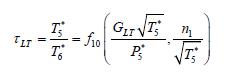(3.1.22)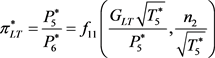( 3.1.23 )

${N}_{eLT}={f}_{12}\left(\frac{{G}_{LT}\sqrt{{T}_{5}^{\ast }}}{{P}_{5}^{\ast }},\frac{{n}_{1}}{\sqrt{{T}_{5}^{\ast }}},{P}_{5}^{\ast },{T}_{5}^{\ast },\beta \right)$ (3.1.24)

${G}_{LT}=\frac{{G}_{LT}\sqrt{{T}_{5}^{\ast }}}{{P}_{5}^{\ast }}\cdot {P}_{5}^{\ast }\cdot \frac{1}{\sqrt{{T}_{5}^{\ast }}}$ (3.1.25)

$\frac{{n}_{3}}{\sqrt{{T}_{6}^{\ast }}}={f}_{13}\left(\frac{{G}_{PT}\sqrt{{T}_{6}^{\ast }}}{{P}_{6}^{\ast }},{\pi }_{PT}^{\ast }\right)$ (3.1.26)

${\tau }_{PT}={f}_{14}\left(\frac{{G}_{PT}\sqrt{{T}_{6}^{\ast }}}{{P}_{6}^{\ast }},{\pi }_{PT}^{\ast }\right)$ (3.1.27)

${N}_{ePT}={f}_{15}\left(\frac{{G}_{PT}\sqrt{{T}_{6}^{\ast }}}{{P}_{6}^{\ast }},\frac{{n}_{3}}{\sqrt{{T}_{6}^{\ast }}},{P}_{6}^{\ast },{T}_{6}^{\ast },\beta \right)$ (3.1.28)

${G}_{PT}=\frac{{G}_{PT}\sqrt{{T}_{6}^{\ast }}}{{P}_{6}^{\ast }}\cdot {P}_{6}^{\ast }\cdot \frac{1}{\sqrt{{T}_{6}^{\ast }}}$ (3.1.29)

${N}_{eN}={f}_{16}\left({n}_{3},{\nu }_{s},\frac{H}{D}\right)$ (3.1.30)(3.1.31)(3.1.32)

3) 联系方程组

${T}_{2}^{\ast }={T}_{1}^{\ast }\cdot {\tau }_{LC}$ (3.1.33)

${P}_{2}^{\ast }={P}_{1}^{\ast }\cdot {\epsilon }_{LC}^{\ast }$ (3.1.34)

${T}_{3}^{\ast }={T}_{1}^{\ast }\cdot {\tau }_{LC}\cdot {\tau }_{HC}$ (3.1.35)

${P}_{3}^{\ast }={P}_{1}^{\ast }\cdot {\epsilon }_{LC}^{\ast }\cdot {\epsilon }_{HC}^{\ast }$ (3.1.36)

${P}_{4}^{\ast }={P}_{1}^{\ast }\cdot {\epsilon }_{LC}^{\ast }\cdot {\epsilon }_{HC}^{\ast }\cdot {\sigma }_{B}$ (3.1.37)

${T}_{4}^{\ast }={f}_{19}\left({T}_{3}^{\ast },\beta ,{\eta }_{B}\right)$ (3.1.38)

${T}_{5}^{\ast }=\frac{{T}_{4}^{\ast }}{{\tau }_{HT}}$ (3.1.39)

${P}_{5}^{\ast }=\frac{{P}_{1}^{\ast }\cdot {\epsilon }_{LC}^{\ast }\cdot {\epsilon }_{HC}^{\ast }\cdot {\sigma }_{B}}{{\epsilon }_{HT}^{\ast }}$ (3.1.40)

${T}_{6}^{\ast }=\frac{{T}_{4}^{\ast }}{{\tau }_{HT}\cdot {\tau }_{HT}}$ (3.1.41)

${P}_{6}^{\ast }=\frac{{P}_{1}^{\ast }\cdot {\epsilon }_{LC}^{\ast }\cdot {\epsilon }_{HC}^{\ast }\cdot {\sigma }_{B}}{{\pi }_{HT}^{\ast }\cdot {\pi }_{LT}^{\ast }}$ (3.1.42)

${T}_{7}^{\ast }=\frac{{T}_{4}^{\ast }}{{\tau }_{HT}\cdot {\tau }_{LT}\cdot {\tau }_{PT}}$ (3.1.43)(3.1.44)

$X=f\left(X,u,\tau \right)$ (3.1.46)

$X={\left({x}_{1},{x}_{2},\cdot \cdot \cdot ,{x}_{n}\right)}^{\text{T}}={\left({\omega }_{1},{\omega }_{2},\cdot \cdot \cdot ,{\omega }_{n}\right)}^{\text{T}}$

u为控制参数，包括油门杆角度、可调节距、可调喷嘴安装角等可调参数，X为n维向量， $\tau$ 为时间坐标。

1) 单机两桨推进主要用于舰艇巡航及以下航速，此时燃气轮机的经济性相对较低，可以通过跨接齿轮箱实现任意一台燃气轮机带动两只螺旋桨工作，在一定程度上改善了燃机在低工况的经济性。

2) 两机两桨推进用于舰艇在巡航与全速之间的特定航速下航行，此工况下左右舷各一台燃机工作。

3) 四机两桨推进模式主要用于舰艇全速航行，长时间在此模式下工作会严重影响燃气轮机及动力装置的使用寿命。

4. 结论

Research on the Mode Calculation and Characteristic Modularization of the Gas Turbine in the Power System[J]. 动力系统与控制, 2019, 08(01): 20-27. https://doi.org/10.12677/DSC.2019.81003

1. 1. Liu, B.J., Zhang, B. and Liu, Y.W. (2015) Investigation of Model Development for Deterministic Correlations Associated with Impeller Diffuser Interactions in Centrifugal Compressors. Science China (Technological Sciences), 58, 499-509.
https://doi.org/10.1007/s11431-015-5766-7

2. 2. Sun, J., Chen, S.Y., Qu, Y.P. and Li, J.F. (2015) Review on Stress Corrosion and Corrosion Fatigue Failure of Centrifugal Compressor Impeller. Chinese Journal of Mechanical Engineering, 28, 217-225.
https://doi.org/10.3901/CJME.2014.1210.178

3. 3. 廖瑛, 梁加红, 姚新宇, 等. 实时仿真理论与支撑技术[M]. 长沙: 国防科技大学出版社, 2012.

4. 4. 康凤举. 舰船仿真技术发展综述[J]. 舰船电子工程, 2004, 24(1): 9-11.

5. 5. 陈华清, 敖晨阳. 舰船动力系统仿真中的柴油机数学模型[J]. 船舶工程, 2000, 22(5): 33-37.

6. 6. 闫东, 梁前超, 等. 某型燃气轮机启动失败的模糊故障树分析[J]. 中国修船, 2015, 28(1): 40-42.

7. 7. 向军, 许光, 杨叔华, 梁前超. 涡轮增压柴油机修后试验及性能仿真[J]. 内燃机与配件, 2016(10): 92-94.

8. 8. Ding, J.M., Wang, Y.S., Ao, C.Y., et al. (2004) Mathematical Modeling and Simulation of Maneuvering for Waterjet-Propelled Catamarans. International Conference of Waterjet Propulsion 4, London: The Royal Institution of Naval Architects, 26-27 May 2004, 1-7.